# Data Analysis and Predictive Models of “1000 Cameras Dataset”

A Python-based Machine Learning Project

# Background Information:

The dataset used in this project can be found on Kaggle (source link: https://www.kaggle.com/crawford/1000-cameras-dataset). The dataset consists of 1038 entries of cameras and their 13 distinct properties, collected by some camera enthusiasts from 1994–2007.

The goal of this project is to first explore the dataset and investigate the relationships among different features in the dataset. Then, I will pick an interesting feature as the target variable, on which I will build multiple types of models to predict its values. After that, I will build and optimize these models accordingly to improve their predicting accuracy while avoiding overfitting. Finally, I will pick the best model for predicting the target variable based on performance.

# Phase 1: Data Preprocessing

Overview of the Dataset

I first took a glimpse at the first five entries of the dataset. This gave me a general idea of what the dataset looks like.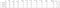The First 5 Entries of the Dataset

I also wanted to know about the detailed variable types of each feature in the dataset. This is an important step for data wrangling later.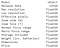Variable Types

From the picture above, we can tell that except for the “Model” variable, all the other variables in the dataset appear to be numerical variables.

Handling Missing Values

It is also important to check if there were any missing values in the dataset that may affect my analysis.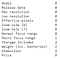Summary of Missing Values

Since there were indeed some missing values, I went ahead and dropped them.

`df.dropna(inplace=True)`

Clean the Data

During my previous exploration of the data, I noticed that some values in the “Weight (inc. batteries)” variable appear to be zero, which does not make sense, as a camera must have at least some weight greater than zero. So, for safety, I will not include these misleading entries of data as well.

`df = df[df['Weight (inc. batteries)'] != 0]`

Further, knowing the specific model type of camera does not necessarily help to model the dataset later, as other features of the camera, such as resolution, focus range, or price, essentially define the model. Nevertheless, the brand of a camera (indicated by the first word in the “Model” variable) may be a valuable variable to look into. Thus, I decided to transform the “Model” variable to a “Brand” variable for better analysis later.

`df['Brand'] = ""df['Brand'] = df.Model.str.split().str.get(0)df.drop(columns=['Model'],inplace=True)`

Additionally, it does not make a lot of sense for the “Release date” variable to be an integer type for modeling, so I converted it to string (essentially treating it as a categorical variable) for better analysis later.

`df['Release date'] = df['Release date'].astype(str)`

Now, after performing a basic preprocessing, let’s dig into the dataset to explore more about these features of cameras and investigate their relationships.

# Phase 2: Data Exploration and Data Wrangling

Histograms of Potentially Interesting Variables

To better determine the target variable for my model, I decided to graph some histograms to see the distribution and spread of some interesting numerical variables in the dataset. Specifically, I picked the “Price” variable and “Weight” variable for visualization.

Both the prices and the weights of the camera dataset are extremely right-skewed, which indicated that they may not be good candidates for a target variable of my model, as this dataset underrepresented cameras of higher prices and weights. Thus, models built based on these data may not have an accurate prediction.

Correlation Matrix

Next, to further visualize the correlation among numerical attributes in this dataset, I plotted a correlation matrix.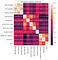Correlation Matrix

From the correlation matrix, I can tell that most numerical variables in this dataset have fairly low correlations with others, with 2 exceptions: resolution vs. effective pixels and dimensions vs. weight. However, these two relationships are not so interesting to explore. Thus, I decided to look more into the categorical variables.

Finalize the Target Variable and the Goal of Modeling

There are only two categorical variables available after data wrangling: the release year of the camera and its brand.

Since there isn’t anything interesting about the release year of the camera, I ultimately decide to choose the “Brand” variable as the target variable for my model.

## Essentially, my model will take in all other attributes of a camera to predict its brand.

Take a Closer Look at the Target Variable

To understand the brands of cameras in this dataset better, I decided to form a statistical and visual summary to categorize the cameras by their brands.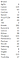Number of Counts of Cameras by Brand

From the pictures above, one potential issue that I found was that some brands only have a little amount of data available. Specifically, Agfa, Contax, JVC, and Sigma all have too few amounts of cameras to be considered significant (Counts < 5). This is problematic, as it will make it more difficult to create an effective model predicting these minor brands of cameras. Thus, to reduce the biases of my predictive model later, I chose to remove the insignificant data of these four brands.

`df.drop(df[(df['Brand'] == 'Agfa')].index, inplace=True)df.drop(df[(df['Brand'] == 'Contax')].index, inplace=True)df.drop(df[(df['Brand'] == 'JVC')].index, inplace=True)df.drop(df[(df['Brand'] == 'Sigma')].index, inplace=True)df.reset_index(drop=True, inplace=True)`

Now that I’ve had a clear understanding of the dataset, it is time for modeling!

# Phase 3: Prepare the Data for Modeling

Type of Models

First, it is important to decide what kind of models to use for predicting the brands of cameras based on other features of cameras.

Since my target variable “Brand” is a categorical variable, modeling methods such as linear regression are clearly not applicable. Further, since my target variable “Brand” is not a binary variable (as it contains several distinctive values), logistical regression is also not appropriate. Thus, I will focus on training my model using methods that are suitable for classification.

Specifically, I will use 3 different methods for modeling in this project: Decision Tree, Bagging, and Ada Boosting.

Convert Categorical Variables into Dummy Variables

First, for my model’s classification to work properly, I have to convert the categorical variable “Release date” into dummy variables.

`df = pd.get_dummies(df, columns=['Release date'])`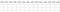Dummy Variables of Release Date

Normalize the Dataset

As you can see from the statistical summary table below, the ranges of the features of the dataset are not the same. This may cause a problem. A small change in a feature might not affect the other. To address this problem, I normalized the ranges of the features to a uniform standard of 0–1.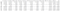Statistical Summary Table of the features

The first 5 entries of the dataset after normalization looked like this: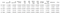Normalized Numerical Variables

Partition the data into training and testing sets

I then split the dataset into two parts: 70% of data for training the model and 30% of data for testing the performance of my model.

`from sklearn.model_selection import train_test_splitX = df.iloc[:, 1:]y = df.iloc[:, 0]X_train, X_test, y_train, y_test = train_test_split(X, y, test_size=0.3, random_state=2021, stratify=y)`

Note: I intentionally added the “stratify=y” parameter, as the data of my target variable “Brand” is unbalanced. “stratify=y” parameter helps reduce biases of the model by preserving the brand proportion within the training and testing set to better form the model.

I then take a look at the shape of my training and testing sets to make sure that they are in the 70:30 proportion.

`print(X_train.shape)print(X_test.shape)print(y_train.shape)print(y_test.shape)`

The proportion looks good! Now let’s dig into the details of constructing the models.

# Phase 4.1: Decision Tree Modeling

Introduction

Decision Tree Modeling is a supervised classification algorithm that is used when the target variable is a categorical variable and the predictor variables are either categorical or numerical. It utilizes a set of if-then rules, represented as a tree, for classification. The visual output of the model makes it easier to understand and implement compared to other predictive models.

Fit the training data to a classification tree

`from sklearn.tree import DecisionTreeClassifierdt = DecisionTreeClassifier(criterion='entropy',max_depth=6,random_state=2021)dt.fit(X_train, y_train)`

Note: For code reproduction purposes, all of the models’ random states are manually set to be 2021.

Feature Importance

After fitting the data, I’m curious to see the most important features that determine the brand of a camera. So I will go ahead and calculate the Top-5 most important features in the decision tree model.

`imp=pd.DataFrame(zip(X_train.columns, dt.feature_importances_), columns=['Feature','Importance'])imp.sort_values(by='Importance', ascending=False, inplace=True)print(imp.head())`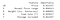Top 5 Important Features of Decision Tree Model

From the table above, It is clear that price is the most important feature in determining the brand of a camera, capturing more than 80% importance.

This result makes sense, as different camera brands, depending on their various company size, brand influence, and customer bases, may result in very different pricing strategies for their cameras. For example, famous brands such as Sony, Canon, and Nixon may have more advanced and professional cameras selling for more expensive prices than some small, unpopular brands. Thus, knowing the price of a camera largely helps predict its brand, based on the decision tree model.

Model Visualization

Let’s also take a look at the confusion matrix of the Decision Tree Model.

`from sklearn import metricsmetrics.plot_confusion_matrix(dt, X_test, y_test)plt.xticks(rotation=90)plt.title("Decision Tree Confusion Matrix")plt.show()`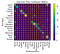Decision Tree Confusion Matrix

Since it is a decision tree model, I also get the advantage of actually seeing how each feature is classified by plotting a classification tree.

`from sklearn import treeplt.figure(2)fn = X.columnscn = y.unique()Tree = tree.plot_tree(dt, feature_names=fn, class_names=cn, filled=True)plt.show()`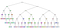The Classification Tree

From the classification tree above, I can tell that the decision tree model created is fairly complex. This makes sense, because in our model, there are 12 distinctive features that altogether determining the brand of a camera. In fact, since we only set “max_depth=6”, which is the maximum depth allowed for expansion of the tree, our model may even seem to be oversimplistic and potentially underfitting.

Accuracy Score

To see how our model actually performs, I used this model on the testing set to get the accuracy score. The resulting testing accuracy is 69.97%. This is not a very ideal accuracy, which further indicates the potential issue of underfitting of the model.

Model Optimization

To optimize the model, I used GridSearch Cross-Validation to tune the model’s parameters. Cross-validation over the grid of hyperparameters will find the combination of hyperparameters that have the lowest test error. Thus, I will find the set of parameters with the least amount of overfitting and with the optimized performance.

`dt_param = {'max_depth':range(5,50), 'random_state':,'criterion': ['gini', 'entropy']}dt_grandsearch = GridSearchCV(DecisionTreeClassifier(), dt_param, n_jobs=5)# Find the optimized decision tree modeldt_grandsearch.fit(X_train,y_train)`

Specifically, I’m curious to find the best max depth for my decision tree within the range of 5–50. I also want to know whether the “entropy” criterion is a better option than the “gini” criterion.

Result:

It turns out that the “entropy” criterion is indeed a better option that gives higher accuracy. Also, instead of setting the max_depth parameter to 6, we should change it to 12 for better performance of the model.

Re-train the Model Using Optimal Parameters

Then, I used max_depth = 12 to train the decision tree model again.

The new accuracy on the testing partition is now 82.78%, a huge leap from before, which proves that the previous model is overly generalizing the data and is underfitting.

To make sure there is no potential overfitting of the new model, I also compare the accuracy score of the model on the testing partition to that on the training partition. Since there is no huge difference between the two, and since the model has high accuracy in predicting the testing set, I concluded that there is no significant overfitting.

I also graph the classification tree for the new model: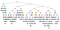The Classification Tree for Optimized Model

It appears more complicated than before, which further confirms the issue of underfitting of the previous model.

# Phase 4.2 Bagging Modeling

Introduction

Bagging Modeling essentially tries to fits base classifiers each on random subsets of the original dataset and then aggregate their individual predictions to form a final prediction. Such a meta-estimator can typically be used as a way to reduce the variance of a black-box estimator (e.g., a decision tree), by introducing randomization into its construction procedure and then making an ensemble out of it.

Fit the training data to a bagging model

I decided to choose the already optimized decision tree model to serve as the base estimator for my bagging model. Theoretically, bagging modeling, as a more advanced classifier, should perform better than my decision tree model, as it introduces randomization to reduce the variance of my decision tree model.

`model_bagging = BaggingClassifier(DecisionTreeClassifier(criterion='entropy',max_depth=12,random_state = 2021),random_state = 2021,n_estimators=50)model_bagging.fit(X_train, y_train)`

Feature Importance

Since Bagging Classifier is designed to be used with potentially many base estimators, so there is no method of feature importance implemented. Nevertheless, I will take a look at the important features again and compare feature importance among different models in the AdaBoost section below.

Model Visualization: Confusion Matrix

Accuracy Score

Using a similar method as mentioned in the decision tree modeling, the accuracy score for the bagging model on the testing set is 84.11%. This already surpassed the decision tree model. It confirms our theoretical hypothesis that the bagging model will perform better, as it adds randomization onto the decision tree (base estimator) to reduce variance and strengthen performance.

Model Optimization

Again to optimize the model, I used GridSearch Cross-Validation to tune the model’s parameters. This time, I tested on whether allowing bootstrapping (samples drawn with replacement) will benefit our performance. I also picked multiple n_estimator values (number of base estimators in the ensemble) to see which one performs the best.

`bag_param = {'bootstrap': [True, False],'bootstrap_features': [True, False],'n_estimators': [50,55,60],'random_state':}bag_grandsearch = GridSearchCV(BaggingClassifier(base_estimator=DecisionTreeClassifier(criterion='entropy',max_depth=12,random_state = 2021)),param_grid=bag_param, n_jobs=5)# Find the optimized Bagging modelbag_grandsearch.fit(X_train,y_train)`

Note: Since bagging is extremely computationally expensive, I only picked three n_estimators: 50, 55, 60. While it is not perfect, it does give us a general idea of if we should use smaller or bigger n_estimators in this case.

Result:

Comparing to my original model, the optimized model suggests using parameters of n_estimators=55, and using bootstrapping during modeling.

Re-train the Model Using Optimal Parameters

Thus, I trained the bagging model again using n_estimators=55, bootstrap=True, bootstrap_features=True accordingly to optimize the model.

The new accuracy on the testing partition is now 89.07%, which is also significantly higher than before.

To make sure there is no potential overfitting of the new model, I again compared the accuracy score of the model on the testing partition to that on the training partition. Since there is no huge difference between the two, and since the model has high accuracy in predicting the testing set, I concluded that there is no significant overfitting.

# Phase 4.3 AdaBoost Modeling

Introduction

AdaBoost Modeling begins by fitting a classifier on the original dataset and then fits additional copies of the classifier on the same dataset but where the weights of incorrectly classified instances are adjusted such that subsequent classifiers focus more on difficult cases. Ideally, it will reduce the biases of the base estimator and increase modeling accuracy.

Fit the training data to an AdaBoost model

Again, I choose the already optimized decision tree model to serve as the base estimator. Theoretically, the AdaBoost model should also perform better than my decision tree model, as it reduces the biases of the decision tree model by handling incorrectly classified instances.

`base_est = DecisionTreeClassifier(criterion='entropy',max_depth=12,random_state = 2021)ada_boost = AdaBoostClassifier(base_est, n_estimators=50, random_state=2021, learning_rate=0.05)ada_boost.fit(X_train, y_train)`

Feature Importance

Using a similar method mentioned in the decision tree modeling, I get the following result:

The feature importance for the price is slightly lowered, due to the weights adjusted on handling difficult cases by the AdaBoost Modeling. Yet, Price still remains a very overwhelming feature that determines the brand of a camera.

Model Visualization: Confusion Matrix

Accuracy Score

Using a similar method as mentioned before, the accuracy score for the AdaBoost model on the testing set is 85.76%. This already surpassed the decision tree model. It confirms our theoretical hypothesis that the AdaBoost model will perform better than the decision tree model, as it reduces the biases of the decision tree model by handling incorrectly classified instances.

Model Optimization

To optimize the model, This time, I used GridSearch Cross-Validation to tune n_estimator values (number of base estimators in the ensemble) and learning_rate (weight applied to each classifier at each boosting iteration) to see get the optimized parameters.

`boosting_param = { 'n_estimators': [50,55,60],'random_state':,'learning_rate': [0.01,0.02,0.03,0.04,0.05,0.06,0.07]}boost_grandsearch = GridSearchCV(AdaBoostClassifier(base_estimator=DecisionTreeClassifier(criterion='entropy',max_depth=12,random_state = 2021)),param_grid=boosting_param, n_jobs=5)# Find the optimized Boosting modelboost_grandsearch.fit(X_train,y_train)`

Result:

Comparing to my original model, the optimized model suggests using parameters of a smaller learning rate (a regularization parameter) when training my model. This result shows that my original AdaBoost model is overfitting so that a smaller learning rate is suggested by GridSearch to perform regularization.

Re-train the Model Using Optimal Parameters

Thus, I trained the bagging model again using learning_rate=0.01 accordingly to optimize the model with regularization.

The new accuracy on the testing partition is now 84.44%, which is a little bit lower than the original model, preventing the AdaBoost model from overfitting.

# Phase 5: Performance Summary

Model Comparison

Let’s now compare the statistics of three optimized models:

a. Decision Tree Model:

• The mean cross-validated score for the Optimized model: 0.7721479229989867
• Accuracy score on the testing partition: 0.8278145695364238

b. Bagging Model (with Decision Tree as base estimator):

• The mean cross-validated score for the Optimized model: 0.8618642350557245
• Accuracy score on the testing partition: 0.890728476821192

c. AdaBoost Model (with Decision Tree as base estimator):

• The mean cross-validated score for the Optimized model: 0.7721479229989867
• Accuracy score on the testing partition: 0.8443708609271523

It is obvious that the Bagging Model appears to have the best performance with the highest accuracy!

Benchmark Model & Baseline Accuracy

Finally, let’s use the DummyClassifier to calculate the baseline accuracy score for our models.

`from sklearn.dummy import DummyClassifier# Constant Strategydummy_clf = DummyClassifier(strategy='most_frequent')dummy_clf.fit(X_train, y_train)dummy_y_pred = dummy_clf.predict(X_test)print("The baseline accuracy is", accuracy_score(y_test, dummy_y_pred))`

In this case, I used the “most_frequent” strategy for my DummyClassifier, as it appears to have the highest accuracy score comparing to all the other strategies (i.e. “Constant”, “Uniform”, and “Stratified”). Yet, due to the great variance of data, the baseline accuracy result is extremely low: 11.92%. All of our models have accuracy well surpassed this benchmark!

# Phase 6: Conclusion

1. Out of the three modeling methods I used, the Bagging Classifier appears to have the best performance on the camera dataset. Thus, using the optimized bagging model, we may fairly predict the brand of a camera by inputting many other features such as camera price, weight, dimension, etc.
2. The Price of a camera is the most important feature in predicting the camera’s brand. One potential reason is that different camera brands may have drastically different pricing strategies based on their various company size, brand influence, and customer bases.
3. The dataset only contains 1000ish entries, which causes the distributions of the target variable and various features skewed. Thus, the dataset may not be representative of the real-time camera market. Consequently, the models trained based on this dataset may result in lower accuracy in real-time prediction than expected.

The End!

For questions, concerns, and comments for improvement, please email me:

johnny_geng@foxmail.com